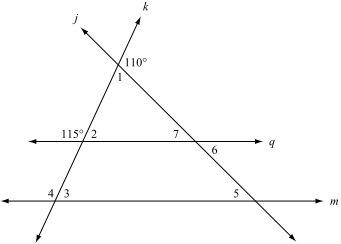# Parallel lines

## What is Parallel lines

### Parallel lines are the subject of Euclid‘s parallel postulate. Parallelism is primarily a property of affine geometries and Euclidean geometry is a special instance of this type of geometry. In some other geometries, such as hyperbolic geometry, lines can have analogous properties that are referred to as parallelism.## What is interior angles

Internal and External angles

### If every internal angle of a simple polygon is less than 180°, the polygon is called convex.## What is Triangle postulate

### It was unknown for a long time whether other geometries exist, where this sum is different. The influence of this problem on mathematics was particularly strong during the 19th century. Ultimately, the answer was proven to be positive: in other spaces (geometries) this sum can be greater or lesser, but it then must depend on the triangle. Its difference from 180° is a case of angular defect and serves as an important distinction for geometric systems.